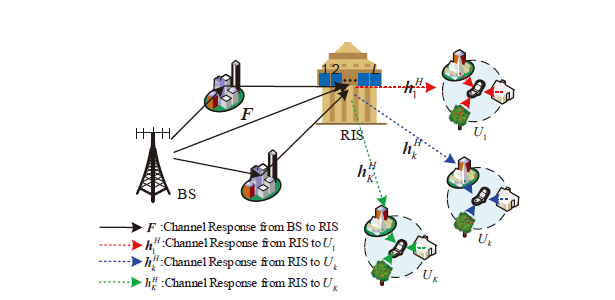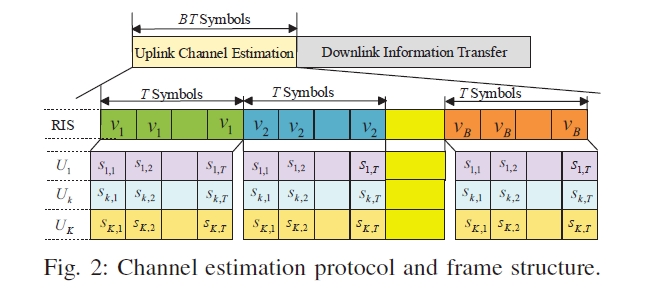# 文章贡献

• 作者指出， 由于基站与反射面往往安置在相近高度，因此其间的散射非常少， 即信道径数很有限。 因此，智能反射面系统的信道有类似于毫米波信道的稀疏性。 但是，由于智能反射面的信道由两部分组成，因此他的稀疏性又有别于普通的毫米波信道， 作者称之为 row-column-block sparse。 这个后面后细说。
• 基于这一特殊的稀疏性， 作者针对性地提出了一种压缩感知算法， 可以相比传统方法更好的恢复信道。
• 由于变量之间的耦合，本文采用了一种交替迭代优化的算法（alternating optimizaiton），并且分析了其收敛性。

# 系统模型• $K$: 用户数
• $M$: 基站天线数 （假定用户为单天线）
• $L$: 智能反射面反射元件数
• $U_1, ..., U_k$, 用户$1$,…用户$k$
• $\mathbf{F}$: $L \times M$, 基站到反射面的信道
• $\mathbf{h}^H_k$: $1 \times L$, 反射面到$k$用户的信道
• $\mathbf{V}_o = \mathbf{diag}(\mathbf{v}_0)$$L \times L$的对角阵， 表示反射面对入射信道的处理（幅度和相位的调整）。本文中认为只有相位的调整。
• 整个信道可表示为： $\mathbf{h}^H_k \mathbf{V}_o\mathbf{F}$

$G_{k}=\operatorname{diag}\left(h_{k}^{H}\right) F \in \mathbb{C}^{L \times M}$

# 估计协议\begin{aligned} \boldsymbol{Y}_{b} &=\sum_{k=1}^{K} \boldsymbol{F}^{H} \operatorname{diag}\left(\boldsymbol{v}_{b}\right) \boldsymbol{h}_{k} \boldsymbol{s}_{k}^{H}+\boldsymbol{U}_{b} \\ & \stackrel{(\mathrm{a})}{=} \sum_{k=1}^{K} \boldsymbol{G}_{k}^{H} \boldsymbol{v}_{b} \boldsymbol{s}_{k}^{H}+\boldsymbol{U}_{b} \end{aligned}

# 最小二乘（LS）估计

$\tilde{\boldsymbol{y}}_{b, k} \triangleq \frac{1}{P T} \boldsymbol{Y}_{b} \boldsymbol{s}_{k}=\boldsymbol{G}_{k}^{H} \boldsymbol{v}_{b}+\boldsymbol{u}_{b, k}$

$\tilde{\boldsymbol{Y}}_{k}=\boldsymbol{G}_{k}^{H} \boldsymbol{V}+\tilde{\boldsymbol{U}}_{k}$

$\hat{G}_{k}=\left(\tilde{\boldsymbol{Y}}_{k} \boldsymbol{V}^{\dagger}\right)^{H}$

# 信道的稀疏表示

©️2019 CSDN 皮肤主题: 大白 设计师: CSDN官方博客